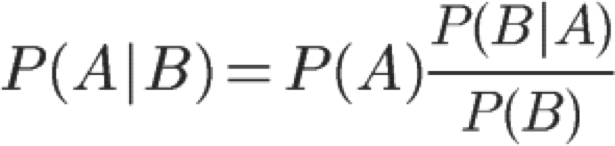# 数学知识——概率论2:聊聊贝叶斯

P(B|A) = P(AB) / P(A)

P(B|A) * P(A) = P(AB)

P(AB)=P(BA)=P(A|B)*P(B)

P(A|B)P(B) = P(B|A)P(A)

P(A|B) = P(B|A)P(A) / P(B)